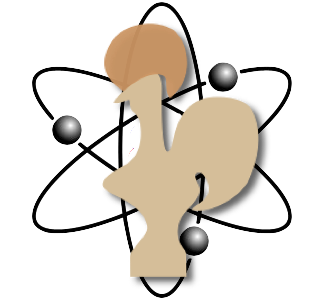# POPL 2020: Verified Quantum Computing

Robert Rand
with Kesha Hietala and Kartik Singhal

## Introduction

This tutorial will introduce quantum computing to a programming languages audience through use of the Coq proof assistant. Beyond introducing attendees to the basics of quantum computing, it will introduce a simple quantum programming language, called SQIR, and demonstrate how to verify the correctness of quantum programs.

Popular presentations of quantum mechanics often portray quantum mechanics as “weird” or “spooky” and fundamentally difficult for laypeople, or even mathematicians and physicists, to wrap their heads around. We will take the opposite approach, explaining quantum computing in terms of a simple mathematical theory that we embed in the Coq proof assistant, relying on nothing but Coq’s real numbers and a lightweight formalization of complex numbers and matrix algebra. This will allow attendees to play with quantum systems, familiarizing themselves with their advantages and limitations.

The first half of the tutorial (Qubit.v and Multiqubit.v) will focus on the basics of quantum computing. In particular, it will address the following questions:

• What are qubits and how do we represent them?
• What are superposition and entanglement?
• What operations are valid in a quantum context?
• How do we measure qubits and mathematically represent the outcomes?

The second half of the tutorial (SQIMP.v) will turn towards subjects of particular interest to POPL attendees: How do we program quantum computers and how do we prove that these programs behave as desired? To that end, we will begin by introducing the small quantum language SQIR, which features in ongoing work as a target for the VOQC compiler. SQIR is a deliberately low-level language, designed for optimization and verification. It allows users to apply basic quantum operations (unitary gates and branching measurement) to a fixed set of qubit registers, the size of which is specified in the type of the program.

We will demonstrate how to program in sqir and how to prove the correctness of SQIR programs, focusing on textbook quantum algorithms like Deutsch’s algorithm and quantum teleportation. This will help us convey the power of quantum computation while giving users a taste of formal verification in the quantum setting.

This tutorial will assume basic knowledge of the Coq proof assistant. No background in quantum computing will be assumed.